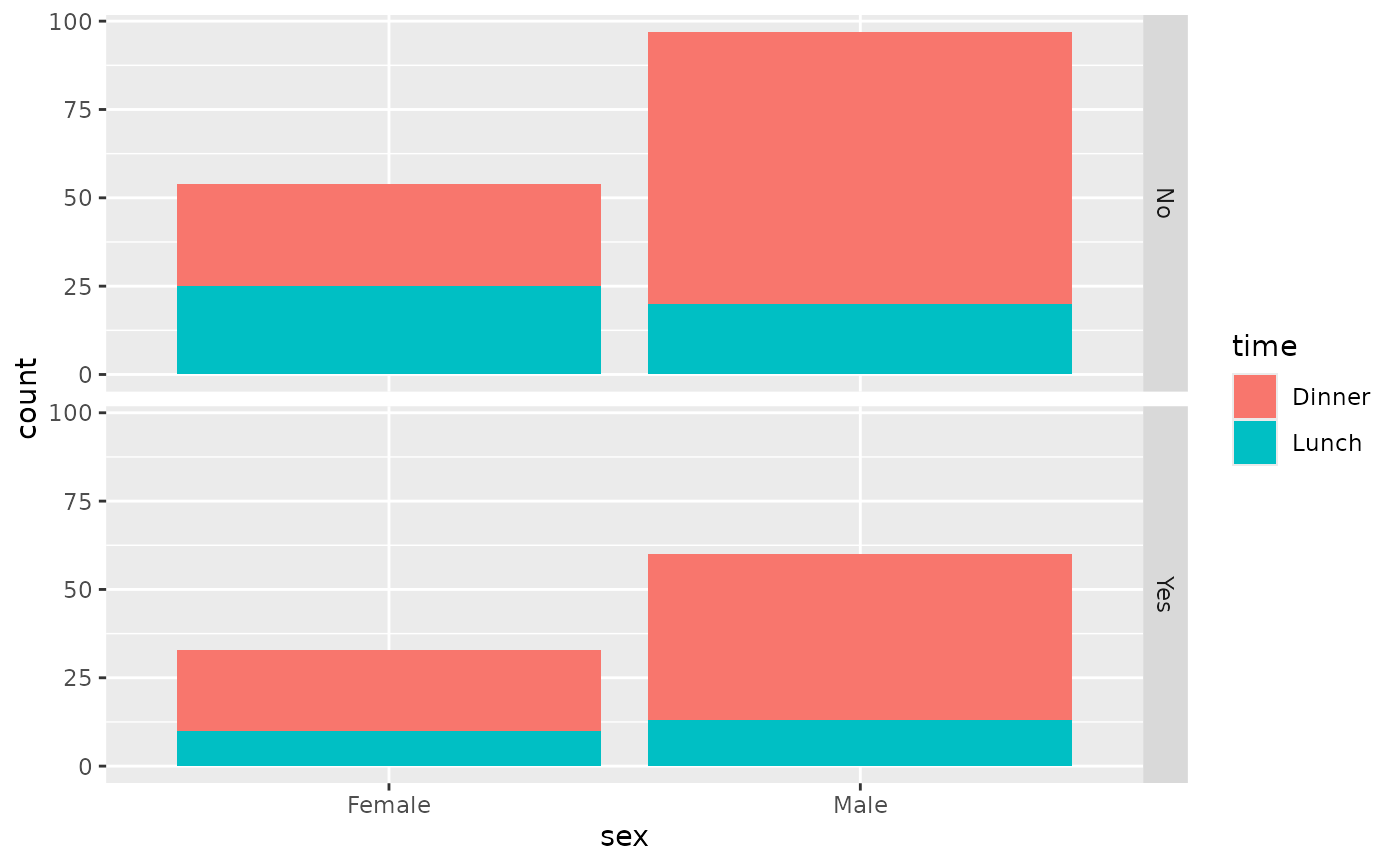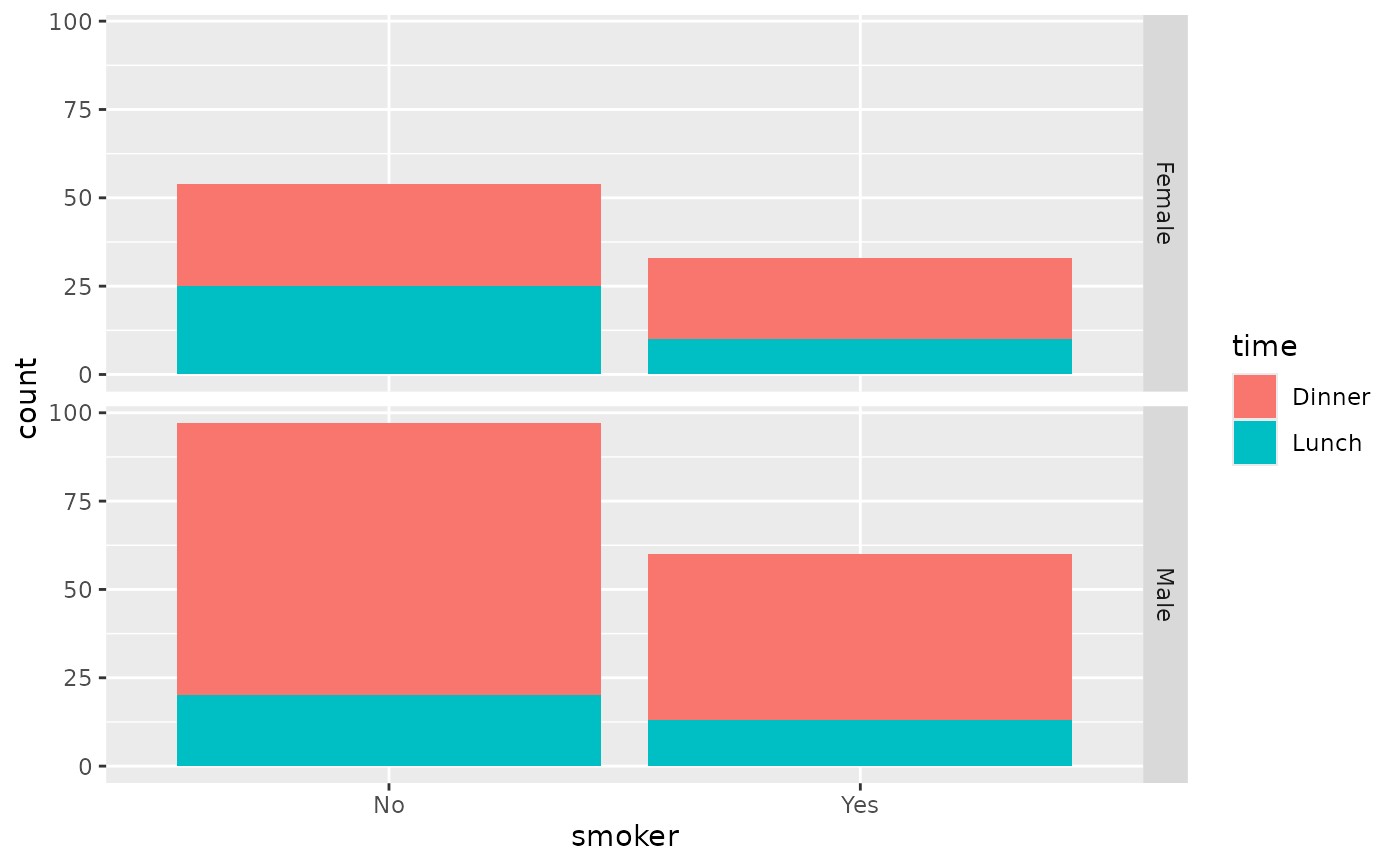X variables are plotted using geom_bar and are faceted by the Y variable.

ggally_facetbar(data, mapping, ...)

## Arguments

data data set using aesthetics being used other arguments are sent to geom_bar

Barret Schloerke

## Examples

# Small function to display plots only if it's interactive
p_ <- GGally::print_if_interactive

data(tips, package = "reshape")
p_(ggally_facetbar(tips, ggplot2::aes(x = sex, y = smoker, fill = time)))p_(ggally_facetbar(tips, ggplot2::aes(x = smoker, y = sex, fill = time)))